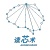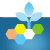We consider the weakly supervised binary classification problem where the labels are randomly flipped with probability $1- {\alpha}$. Although there exist numerous algorithms for this problem, it remains theoretically unexplored how the statistical accuracies and computational efficiency of these algorithms depend on the degree of supervision, which is quantified by ${\alpha}$. In this paper, we characterize the effect of ${\alpha}$ by establishing the information-theoretic and computational boundaries, namely, the minimax-optimal statistical accuracy that can be achieved by all algorithms, and polynomial-time algorithms under an oracle computational model. For small ${\alpha}$, our result shows a gap between these two boundaries, which represents the computational price of achieving the information-theoretic boundary due to the lack of supervision. Interestingly, we also show that this gap narrows as ${\alpha}$ increases. In other words, having more supervision, i.e., more correct labels, not only improves the optimal statistical accuracy as expected, but also enhances the computational efficiency for achieving such accuracy.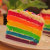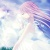The goal of regression and classification methods in supervised learning is to minimize the empirical risk, that is, the expectation of some loss function quantifying the prediction error under the empirical distribution. When facing scarce training data, overfitting is typically mitigated by adding regularization terms to the objective that penalize hypothesis complexity. In this paper we introduce new regularization techniques using ideas from distributionally robust optimization, and we give new probabilistic interpretations to existing techniques. Specifically, we propose to minimize the worst-case expected loss, where the worst case is taken over the ball of all (continuous or discrete) distributions that have a bounded transportation distance from the (discrete) empirical distribution. By choosing the radius of this ball judiciously, we can guarantee that the worst-case expected loss provides an upper confidence bound on the loss on test data, thus offering new generalization bounds. We prove that the resulting regularized learning problems are tractable and can be tractably kernelized for many popular loss functions. We validate our theoretical out-of-sample guarantees through simulated and empirical experiments.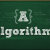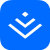【我是这样理解--SVM，不需要繁杂公式的那种！(附代码）】，作者：mantch
链接：
http://t.cn/AiWU1JAh
[向右]导读：1. 讲讲SVM
1.1 一个关于SVM的童话故事
支持向量机（Support Vector Machine，SVM）是众多监督学习方法中十分出色的一种，几乎所有讲述经典机器学习方法的教材都会介绍。关于SVM，流传着...全文【用无监督数据增扩推进半监督学习】《Advancing Semi-supervised Learning with Unsupervised Data Augmentation | Google AI Blog》 http://t.cn/AiW2TzpP paper:《Unsupervised Data Augmentation for Consistency Training》Q Xie, Z Dai, E Hovy, M Luong, Q V. Le [Google Brain] (2019)...全文While unsupervised variational autoencoders (VAE) have become a powerful tool in neuroimage analysis, their application to supervised learning is under-explored. We aim to close this gap by proposing a unified probabilistic model for learning the latent space of imaging data and performing supervised regression. Based on recent advances in learning disentangled representations, the novel generative process explicitly models the conditional distribution of latent representations with respect to the regression target variable. Performing a variational inference procedure on this model leads to joint regularization between the VAE and a neural-network regressor. In predicting the age of 245 subjects from their structural Magnetic Resonance (MR) images, our model is more accurate than state-of-the-art methods when applied to either region-of-interest (ROI) measurements or raw 3D volume images. More importantly, unlike simple feed-forward neural-networks, disentanglement of age in latent representations allows for intuitive interpretation of the structural developmental patterns of the human brain.

Top